## An object is undergoing simple harmonic motion with frequency f = 3.5 Hz and an amplitude of 0.15 m. At t = 0.00 s the object is at x = 0.00

Question

An object is undergoing simple harmonic motion with frequency f = 3.5 Hz and an amplitude of 0.15 m. At t = 0.00 s the object is at x = 0.00 m. How long does it take the object to go from x = 0.00 m to x = 4.00×10−2 m.

in progress 0
2 weeks 2021-08-29T03:07:42+00:00 1 Answers 0 views 0

Time taken by the object is 0.012 s .

Explanation:

Given :

Frequency , f = 3.5 Hz .

Amplitude , A = 0.15 m .

At time t = 0 , x = 0 m.

Since , at time t = 0 , x = 0 m .

Therefore , equation of displacement is :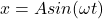…equation 1.

Here ,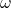is angular frequency and is given by :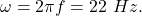We need to find the time at which its displacement is ,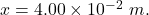Putting all these value in equation 1 we get ,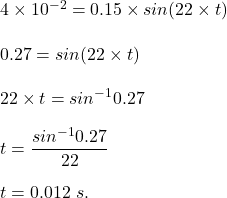Hence , this is the required solution.